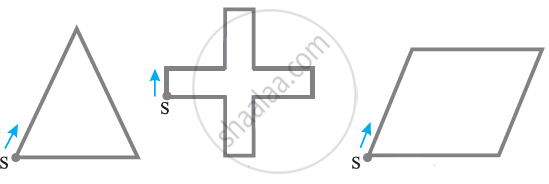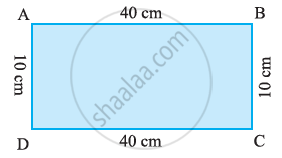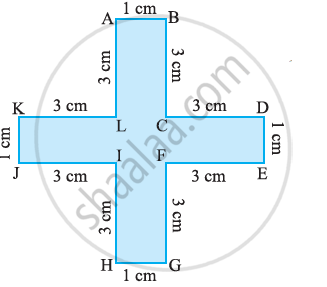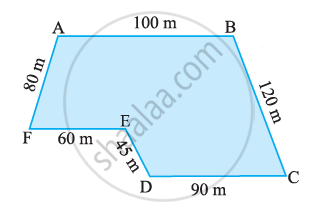# Concept of Perimeter

#### definition

Perimeter: Perimeter is the distance covered along the boundary forming a closed figure when you go round the figure once.

#### formula

Perimeter = Sum of all side of a shape.

# Perimeter:

Look at the following figures, You can make them with a wire or a string.If you start from the point S in each case and move along the line segments then you again reach the point S. You have made a complete round of the shape in each case. The distance covered is equal to the length of wire used to draw the figure. This distance is known as the perimeter of the closed figure. It is the length of the wire needed to form the figures.

Perimeter is the distance covered along the boundary forming a closed figure when you go round the figure once.

### The idea of the perimeter is widely used in our daily life:

• A farmer who wants to fence his field.
• An engineer who plans to build a compound wall on all sides of a house.
• A person preparing a track to conduct sports.

#### Example

Find the perimeter of the following figure:Perimeter = AB + BC + CD + DA

= 40 + 10 + 40 + 10

= 100 cm

#### Example

Find the perimeter of the following figure:Perimeter =AB + BC + CD + DE + EF + FG + GH + HI + IJ + JK + KL + LA

= 1 + 3 + 3 + 1 + 3 + 3 + 1 + 3 + 3 + 1 + 3 + 3.

= 28 cm

#### Example

Find the perimeter of the following figure:Perimeter = AB + BC + CD + DE + EF + FA

= 100 + 120 + 90 + 45 + 60 + 80

= 495 m

If you would like to contribute notes or other learning material, please submit them using the button below.

### Shaalaa.com

Perimeter [00:06:13]
S
0%